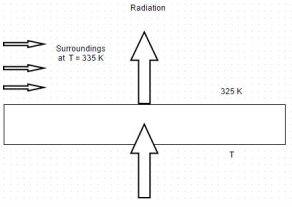# Test: Fourier Equation

## 10 Questions MCQ Test Heat Transfer for Engg. | Test: Fourier Equation

Description
This mock test of Test: Fourier Equation for Chemical Engineering helps you for every Chemical Engineering entrance exam. This contains 10 Multiple Choice Questions for Chemical Engineering Test: Fourier Equation (mcq) to study with solutions a complete question bank. The solved questions answers in this Test: Fourier Equation quiz give you a good mix of easy questions and tough questions. Chemical Engineering students definitely take this Test: Fourier Equation exercise for a better result in the exam. You can find other Test: Fourier Equation extra questions, long questions & short questions for Chemical Engineering on EduRev as well by searching above.
QUESTION: 1

### The famous Fourier series is named after

Solution:

He gave this theory in 1824.

QUESTION: 2

### Fourier law of heat conduction is best represented by

Solution:

According to Fourier law of heat conduction, Q = -k A d t /d x.

QUESTION: 3

### Here are some assumptions that are made for Fourier law. Identify the wrong one

Solution:

It has constant temperature gradient and a linear temperature profile.

QUESTION: 4

Consider the following statements:

The Fourier heat conduction equation
Q = -k A d t /d x
Presumes
ii) Constant value of thermal conductivity
iii) Uniform temperature at the wall surface
iv) One dimensional heat flow

Which of these statements are correct?

Solution:

Thermal conductivity is different for different materials.

QUESTION: 5

The diagram shows heat conduction through a plane wall. The surface temperature is 475 K and it radiates heat to the surroundings at 335 K. If thermal conductivity of the material is 12.5 W/m degree, find the temperature gradient. Let convective coefficient be 80 W/m2 degree and radiation factor is 0.9Solution:

Heat conducted through the plate = convection heat losses + radiation heat losses. So, d t /d x = – 13155/12.5 = – 1052.4 degree Celsius.

QUESTION: 6

Solution:

The ratio d t/d x represents the change in temperature per unit thickness i.e. the temperature gradient. So it represents heat flow in the direction of negative temperature gradient.

QUESTION: 7

Transmission of heat i.e. molecular is smallest in case of

Solution:

In gases atoms are arranged loosely, there is less molecular transmission of heat as compared to solids, liquids and alloys.

QUESTION: 8

Which one is not the unit of thermal conductivity?

Solution:

The unit kcal/m hr K could also be specified as J/m s K or W/m s K and this is actually done while quoting the numerical values of thermal conductivity.

QUESTION: 9

“Thermal conductivity represents the amount of heat conducted across unit area when a temperature difference of one kelvin”. True or false

Solution:

It is across unit area and through unit distance.

QUESTION: 10

Which of the following is the unit of thermal resistance?

Solution:

Thermal resistance is expressed in the unit’s hr degree/kcal, degree/W and s degree/J.